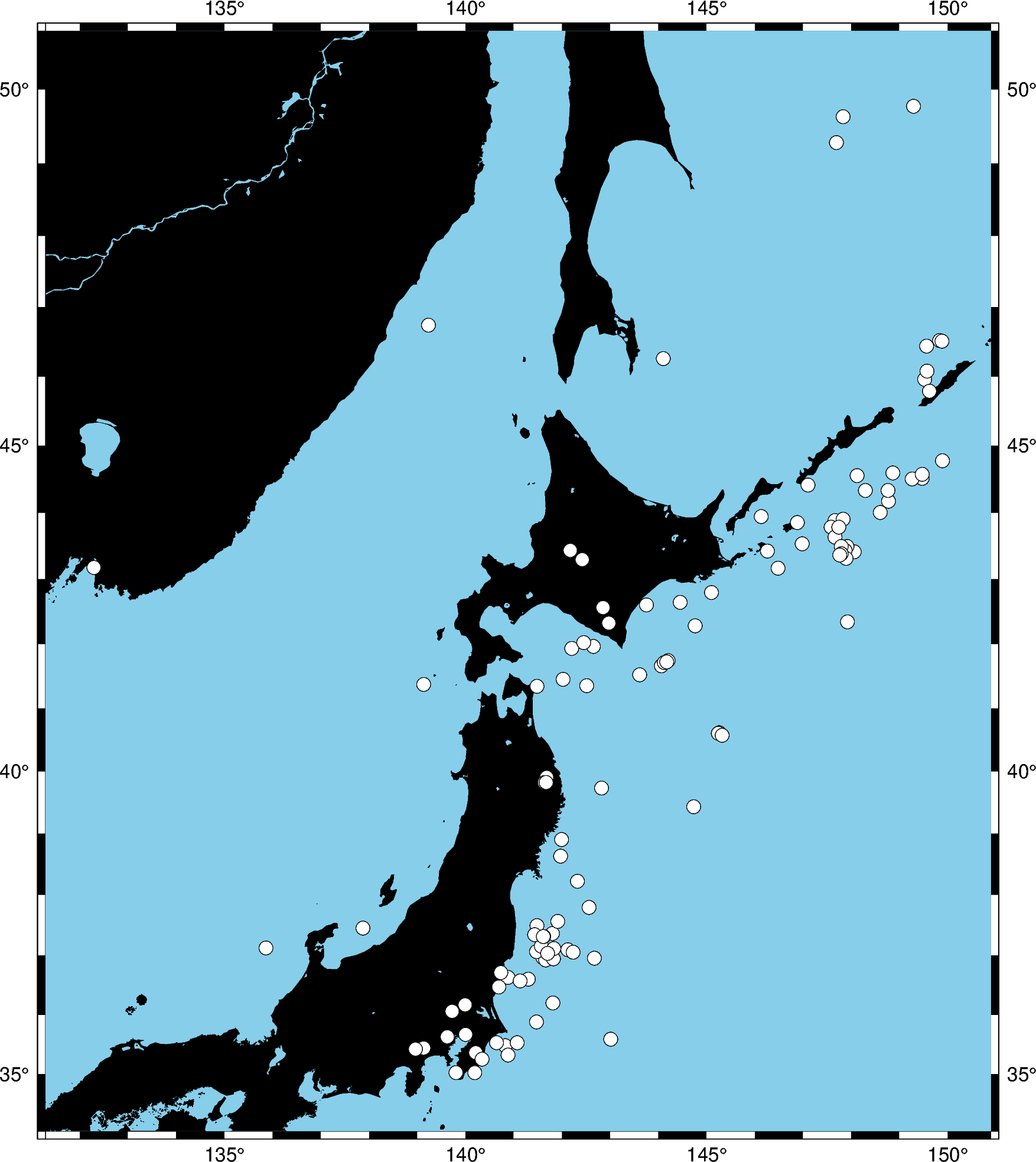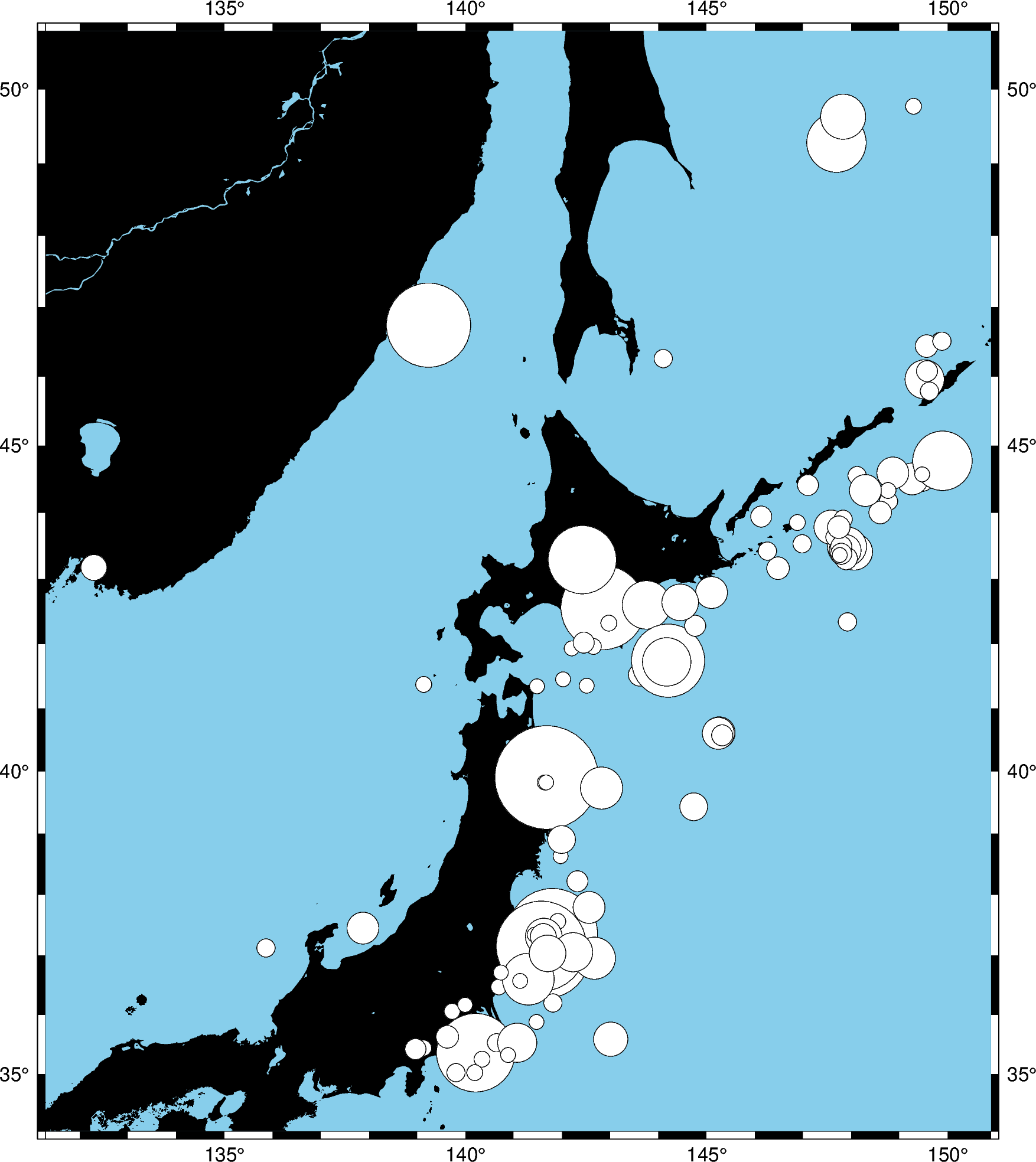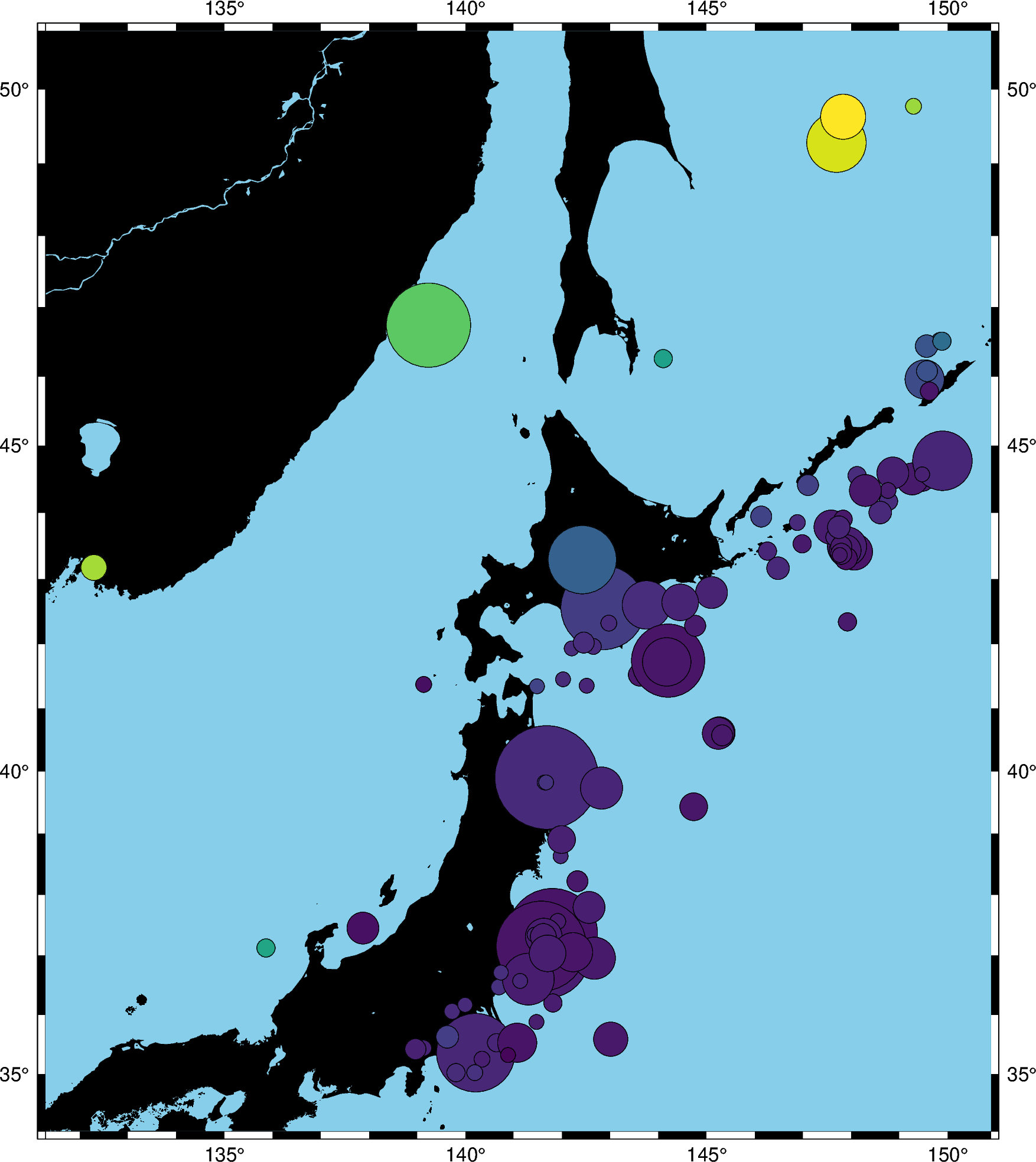# Plotting data points¶

GMT shines when it comes to plotting data on a map. We can use some sample data that is packaged with GMT to try this out. PyGMT provides access to these datasets through the `pygmt.datasets` package. If you don’t have the data files already, they are automatically downloaded and saved to a cache directory the first time you use them (usually `~/.gmt/cache`).

```import pygmt
```

For example, let’s load the sample dataset of tsunami generating earthquakes around Japan (`pygmt.datasets.load_japan_quakes`). The data is loaded as a `pandas.DataFrame`.

```data = pygmt.datasets.load_japan_quakes()

# Set the region for the plot to be slightly larger than the data bounds.
region = [
data.longitude.min() - 1,
data.longitude.max() + 1,
data.latitude.min() - 1,
data.latitude.max() + 1,
]

print(region)
```

Out:

```[131.29, 150.89, 34.02, 50.77]
year  month  day  latitude  longitude  depth_km  magnitude
0  1987      1    4     49.77     149.29       489        4.1
1  1987      1    9     39.90     141.68        67        6.8
2  1987      1    9     39.82     141.64        84        4.0
3  1987      1   14     42.56     142.85       102        6.5
4  1987      1   16     42.79     145.10        54        5.1
```

We’ll use `pygmt.Figure.plot` method to plot circles on the locations of the hypocenters of the earthquakes.

```fig = pygmt.Figure()
fig.basemap(region=region, projection="M8i", frame=True)
fig.coast(land="black", water="skyblue")
fig.plot(x=data.longitude, y=data.latitude, style="c0.3c", color="white", pen="black")
fig.show()
```Out:

```<IPython.core.display.Image object>
```

We used the style `c0.3c` which means “circles of 0.3 centimeter size”. The `pen` attribute controls the outline of the symbols and the `color` controls the fill.

We can map the size of the circles to the earthquake magnitude by passing an array to the `sizes` argument. Because the magnitude is on a logarithmic scale, it helps to show the differences by scaling the values using a power law.

```fig = pygmt.Figure()
fig.basemap(region=region, projection="M8i", frame=True)
fig.coast(land="black", water="skyblue")
fig.plot(
x=data.longitude,
y=data.latitude,
sizes=0.02 * (2 ** data.magnitude),
style="cc",
color="white",
pen="black",
)
fig.show()
```Out:

```<IPython.core.display.Image object>
```

Notice that we didn’t include the size in the `style` argument this time, just the symbol `c` (circles) and the unit `c` (centimeter). So in this case, the sizes will be interpreted as being in centimeters.

We can also map the colors of the markers to the depths by passing an array to the `color` argument and providing a colormap name (`cmap`). We can even use the new matplotlib colormap “viridis”.

```fig = pygmt.Figure()
fig.basemap(region=region, projection="M8i", frame=True)
fig.coast(land="black", water="skyblue")
fig.plot(
x=data.longitude,
y=data.latitude,
sizes=0.02 * 2 ** data.magnitude,
color=data.depth_km / data.depth_km.max(),
cmap="viridis",
style="cc",
pen="black",
)
fig.show()
```Out:

```<IPython.core.display.Image object>
```

Note

We normalize the data values given to `color` because, by default, `plot` can only interpret values between 0 and 1. To use the actual data values, we would need to create a color palette table (CPT) which isn’t implemented yet.

Total running time of the script: ( 0 minutes 3.807 seconds)

Gallery generated by Sphinx-Gallery